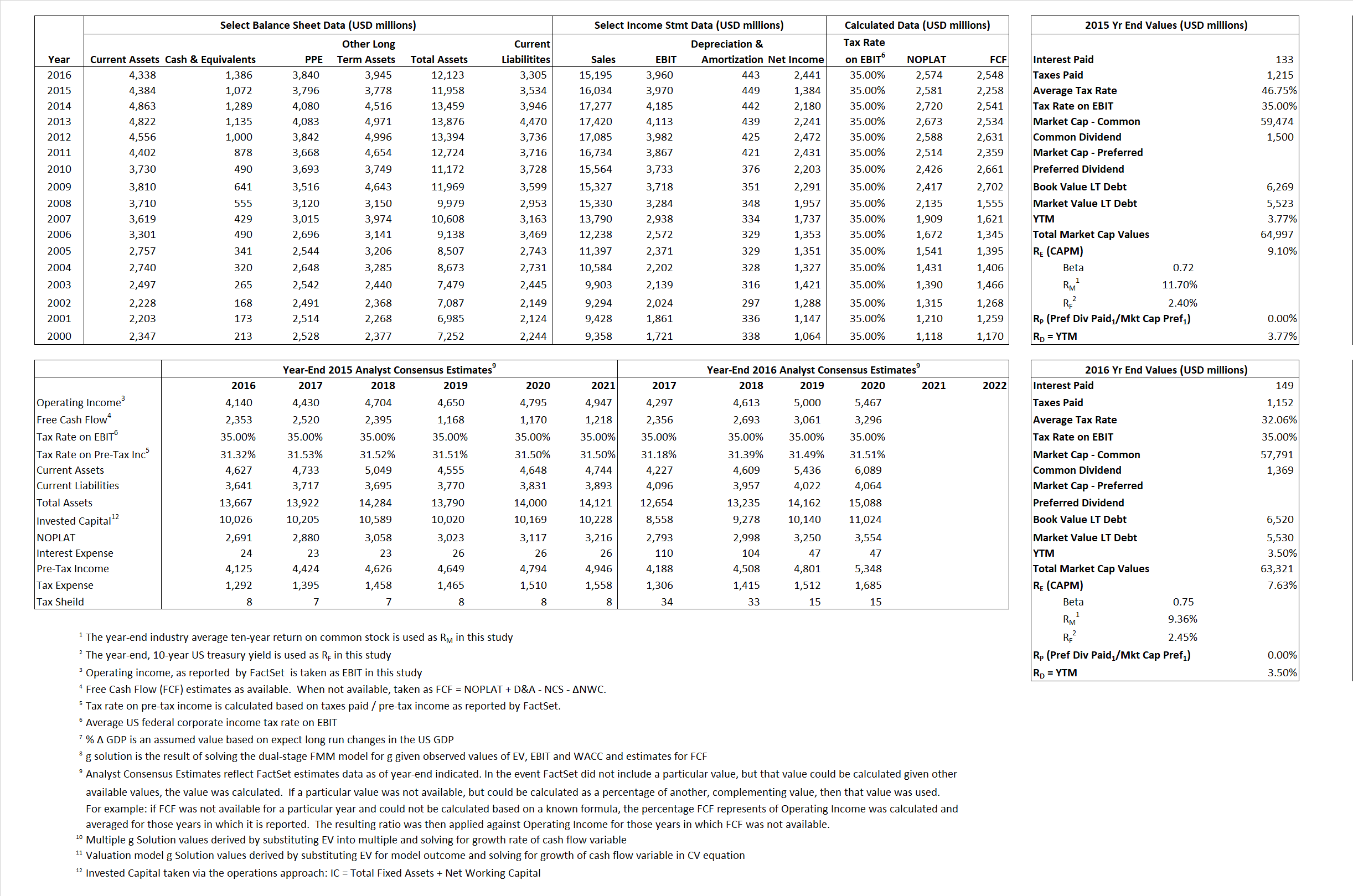# Colgate-Palmolive Company

## Analyst Listing

The following analysts provide coverage for the subject firm as of May 2016:

 Broker Analyst Analyst Email Bernstein Research Ali Dibadj ali.dibadj@bernstein.com CRT Capital Group April Scee ascee@sterneageecrt.com Deutsche Bank Research Bill Schmitz Jr. william.schmitz@db.com Atlantic Equities Charles Eden c.eden@atlantic-equities.com Consumer Edge Research Javier Escalante jescalante@consumeredgeresearch.com William Blair Jon R. Andersen jandersen@williamblair.com Raymond James Joseph Altobello joe.altobello@raymondjames.com First Global Stockbroking Kavita Thomas kavita.thomas@fglobal.com B Riley & Co Linda Bolton Weiser lweiser@brileyco.com Stifel Nicolaus Mark S. Astrachan msastrachan@stifel.com RBC Capital Markets Nik Modi nik.modi@rbccm.com SunTrust Robinson Humphrey William B. Chappell Jr. bill.chappell@suntrust.com

## Primary Input Data## Derived Input Data

### Equational Form

Net Operating Profit Less Adjusted Taxes NOPLAT  2,581 2,574$NOPLAT\, =\, EBIT\, x\, (1 \,-\, Avg \,\,Tax\,\, Rate\,\, on\,\, EBIT)$
Free Cash Flow FCF 2,258 2,548$FCF\,=NOPLAT\,+\,Non-Cash\,Expenses-\Delta NWC\,-\,NCS$
Tax Shield TS 62 48$TS\,=\,Interest\,\,Paid\,\,x\,\, Avg \,\,Tax\,\,Rate\,\, on\,\, Pre-Tax\,\, Income$
Invested Capital IC 8,424 8,818$IC\,=\,Fixed\,\,Operating\,\,Assets\,\,+\,\,Net\,\, Working\,\, Capital$
Return on Invested Capital ROIC 30.63% 29.19%$ROIC\,=\,\frac { NOPLAT }{ IC }$
Net Investment NetInv (640) 837$NetInv\,=\,{ {IC}_{1}}-{{IC}_{0}}+Depreciation$
Investment Rate IR  -24.80%  32.52%$IR\,=\,\frac {NetInv}{NOPLAT}$
Weighted Average Cost of Capital
WACCMarket 8.49%  7.17%$WACC\,=\,\frac { E }{ V } { R }_{ E }\,+\,\frac { P }{ V } { R }_{ P }\,+\,\frac { D }{ V } { R }_{ D }\left( 1- Avg\,\, Tax\,\,Rate\,\,on\,\,Pre-Tax\,\,Income \right)$
WACCBook    6.85%  6.82%
Enterprise value
EVMarket 63,925 61,935$EV\,=\,Market\,\,Cap\,\,Equity\,+\,\,Long\,\,Term\,\,Debt\,-\,Cash$
EVBook    64,671  62,925
Long-Run Growth
g = IR x ROIC
-7.60%   9.49% Long-run growth rates of the income variable are used in the Continuing Value portion of the valuation models.
g = %$\Delta$ GDP     2.50%   2.50%
Margin from Operations M   24.76%  26.06%$M\,\,=\,\,\frac{EBIT}{SALES}$
Depreciation/Amortization Rate D   10.16%  10.06%$D\,\,=\,\,\frac{D+A}{EBITDA}$

## Valuation Multiple Outcomes

The outcomes presented in this study are the result of original input data, derived data, and synthesized inputs.

### model g solution

12/31/2015 12/31/2016 12/31/2015 12/31/2016 12/31/2015 12/31/2016

EV/SALES$\frac {EV}{Sales} \,= \,\frac{ROIC\, -\, g}{ROIC\,(WACC\,-\,g)}\,(1\,-\,T)\,(M)$

3.99  4.08  5.13%  3.52%  6.04%  4.51%

EV/EBITDA$\frac {EV}{EBITDA} \,= \,\frac{ROIC\, -\, g}{ROIC\,(WACC\,-\,g)}\,(1\,-\,T)\,(1\,-\,D)$

14.47 14.07 5.13% 3.52% 6.04% 4.51%

EV/NOPLAT$\frac {EV}{NOPLAT} \,= \,\frac{ROIC\, -\, g}{ROIC\,(WACC\,-\,g)}$

24.77 24.03 5.13% 3.52% 6.04% 4.51%

EV/FCFOPS$\frac {EV}{FCF_{OPS}} \,= \,\frac{ROIC\, -\, g}{ROIC\,(WACC\,-\,g)}\,(1\,-\,T)$

28.31 24.31 5.13% 3.52% 6.04% 4.51%

EV/EBIT$\frac {EV}{EBIT} \,= \,\frac{ROIC\, -\, g}{ROIC\,(WACC\,-\,g)}\,(1\,-\,T)$

16.10 15.64 5.13% 3.52% 6.04% 4.51%

EV/IC$\frac {EV}{IC} \,= \,\frac{ROIC\, -\, g}{WACC\,-\,g}$

7.59 7.02 5.13% 3.52% 6.04% 4.51%